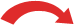# Convert Fractions to Decimals

### The simplest method is to use a calculator.

Just divide the top of the fraction by the bottom, and read off the answer!

### Example: What is 5 8 as a decimal ... ?... get your calculator and type in "5 / 8 ="

### Example: here is what long division of 5 8 looks like:

0.625
8 )5.000
0
5.0
4.8
20
16
40
40
0

In that case we inserted extra zeros and did 5.000 8 to get 0.625

Read the Long Division to Decimal Placespage for more details.

## Another Method

### Yet another method you may like is to follow these steps:

• Step 1: Find a number you can multiply by the bottom of the fraction to make it 10, or 100, or 1000, or any 1 followed by 0s.
• Step 2: Multiply both top and bottom by that number.
• Step 3. Then write down just the top number, putting the decimal point in the correct spot (one space from the right hand side for every zero in the bottom number)

### Example: Convert 3 4 to a Decimal

Step 1: We can multiply 4 by 25 to become 100

Step 2: Multiply top and bottom by 25:

 ×253 4 = 75 100×25

Step 3: Write down 75 with the decimal point 2 spaces from the right (because 100 has 2 zeros);

### Example: Convert 3 16 to a Decimal

Step 1: We have to multiply 16 by 625 to become 10,000

Step 2: Multiply top and bottom by 625:

 ×6253 16 = 1,875 10,000×625

Step 3: Write down 1875 with the decimal point 4 spaces from the right (because 10,000 has 4 zeros);

### Example: Convert 1 3 to a Decimal

Step 1: There is no way to multiply 3 to become 10 or 100 or any "1 followed by 0s", but we can calculate an approximate decimal by choosing to multiply by, say, 333

Step 2: Multiply top and bottom by 333:

 ×3331 3 = 333 999×333

Step 3: Now, 999 is nearly 1,000, so let us write down 333 with the decimal point 3 spaces from the right (because 1,000 has 3 zeros):

Answer = 0.333 (accurate to only 3 decimal places !!)